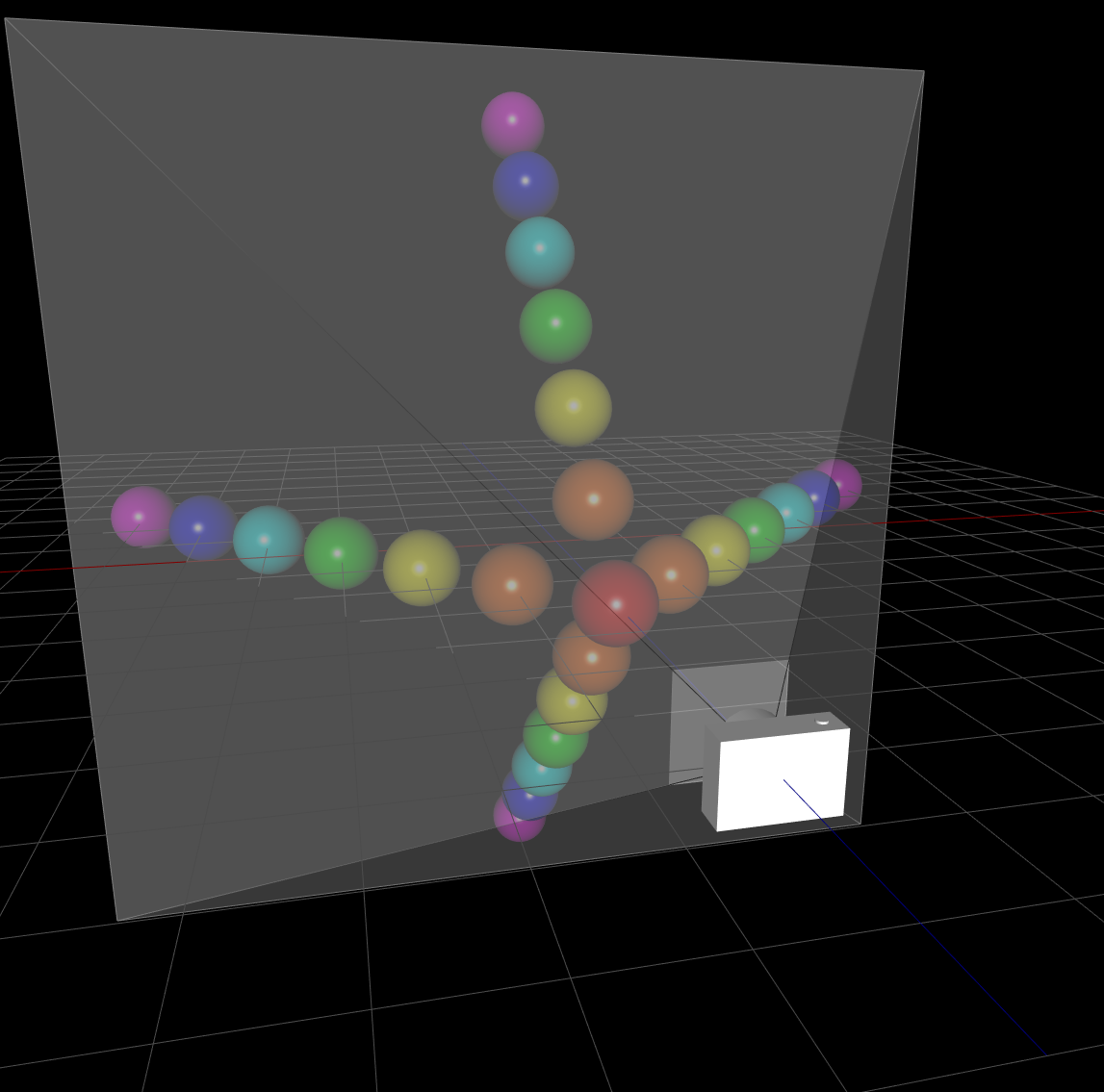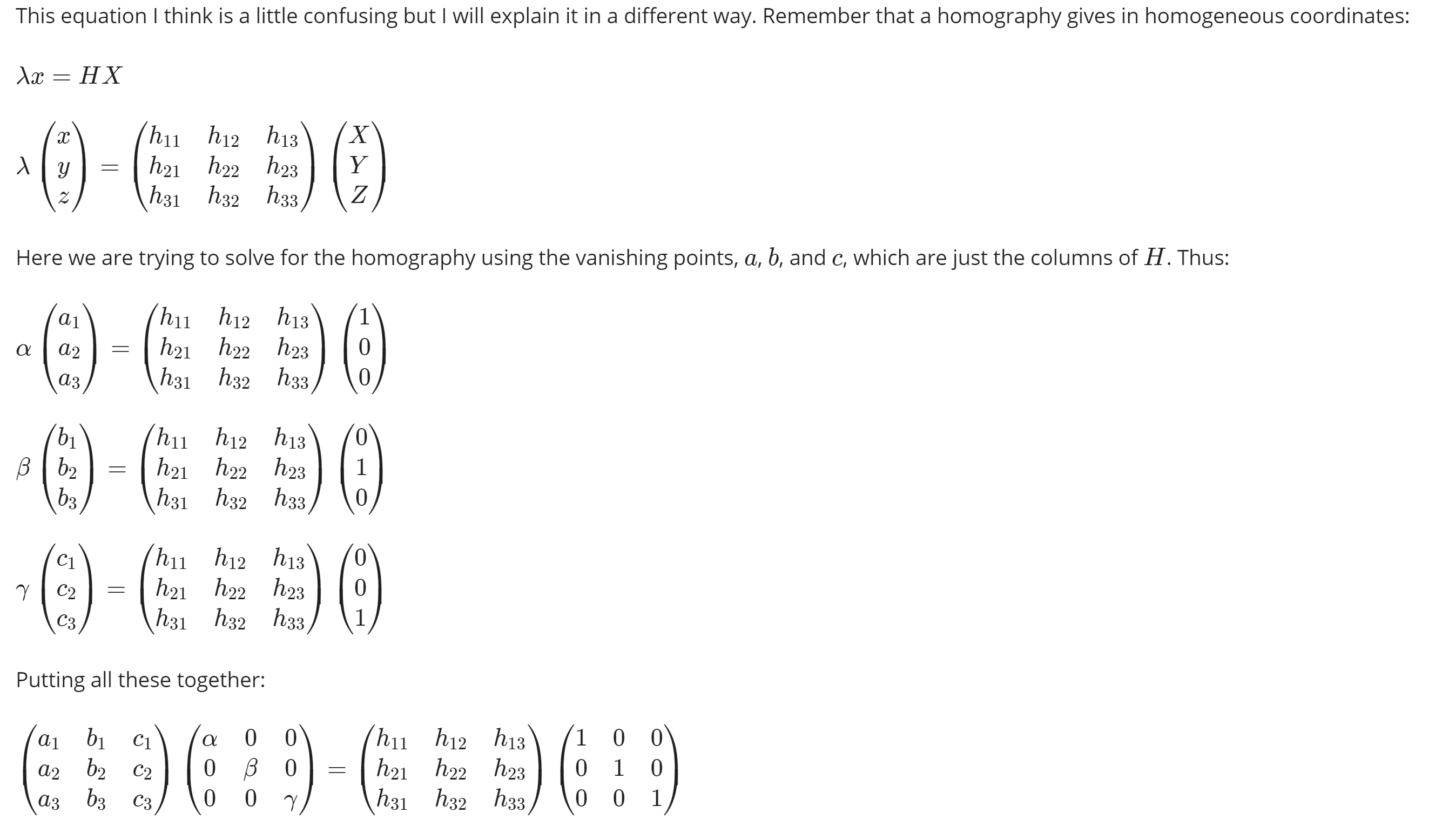# Robotics Perception week2

less than 1 minute read

## Homogeneous Coordinate[齐次坐标]

a combining form appearing in loanwords from Greek, where it meant “same” (homology); on this model, used in the formation of compound words (homomorphic).

## 实际问题

• 对于平面上的两条平行直线 \begin{aligned} l_1 &: AX+BY+C=0\\ l_2 &: AX+BY+D=0\\ \end{aligned}
• 求解时，若$C\neq D$则无解, 若$C=D$, 则$l_1,l_2$重合
• 引入新的维度$w$, 令 \begin{aligned} l_1 &: AX+BY+Cw=0\\ l_2 &: AX+BY+Dw=0\\ \end{aligned}
• 此时存在解$(x,y,0)$, 作为两平行线的交点坐标
• 它的本质，是将分母提出来，避免分母为零误解的情况发生
• 对于$w$不为0时的情况
• 齐次坐标$(x,y,w)$等价于笛卡尔坐标$(\frac{x}{w}, \frac{y}{w})$
• 那么反过来，笛卡尔坐标$(x,y)$对应齐次坐标$(\lambda x,\lambda y,\lambda *1)$
• 我们发现新的坐标系下每个点前的系数阶都是一致的，因此我们称之为齐次坐标
• 更一般的，对于一个$k$次多项式$g(x,y)$,可以通过将$x\rightarrow \frac{x}{z}, y\rightarrow \frac{y}{z}$再乘$z^k$的形式变为齐次形式，即
• $f(x,y,z) = z^kg(\frac{x}{z}, \frac{y}{z})$
• 此时，方程的每一项都已阶数$k$一致的项，这就是Homo的来源

## 齐次坐标的好处

1. 以上可以用$(x,y,0)$表示两个平行线在无穷远处的交点在射影面上的表示2. 直线的齐次表示$(a, b, c)$代表$ax+by+c=0$, 满足所有方程上的点的集合
1. 其几何含义为，以$<a,b,c>$为法向量在3维空间中与投影面($z=Z$)的交线
2. 因此，当$<0,0,c>$表示无法与投影面相交的无穷远处的直线
3. 而$<0,0,0>$无法表示成射线形式，因此在射影空间$\mathbb{P}^2$为$\mathbb{R}^3-(0,0,0)$，即去掉原点的三维实数空间
3. 点的齐次表示, $\mathbf{x} = [x,y,1]^T$, $\mathbf{x}^T[a,b,c]=0$表示满足在线上的点
1. 有，点$\mathbf{x}$在直线$I$上，当且仅当$\mathbf{x}^T\mathbf{I}=0$
2. 直线交点，即，两个直线叉乘后的向量表示
3. 即$\mathbf{x}$满足$\mathbf{I_1}^t\mathbf{x}=\mathbf{I_2}^t\mathbf{x}=0$
4. $\mathbf{x}=\mathbf{I_1}\times \mathbf{I_2}$
4. 过两点的直线
1. $\mathbf{I}=\mathbf{x_1}\times\mathbf{x_2}$

## PerceptionCategories:

Updated: